# Ch 15 Web

Unionville High School
10 de Nov de 2009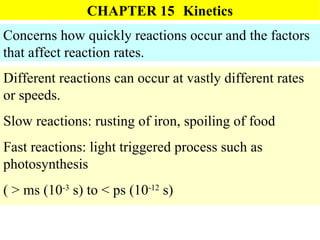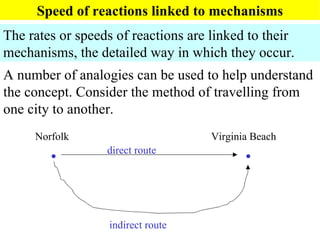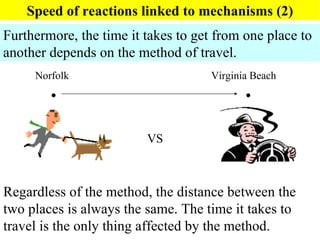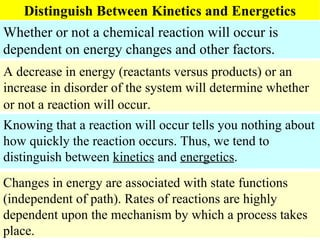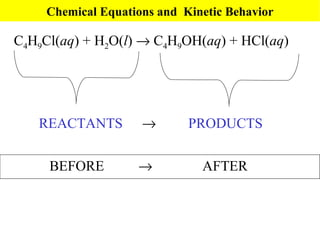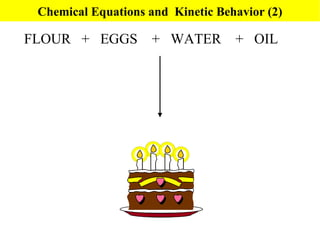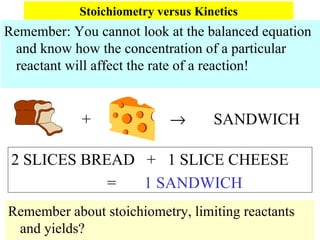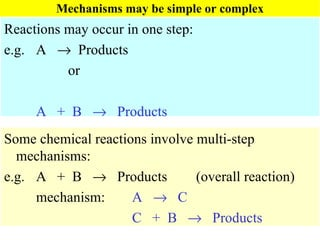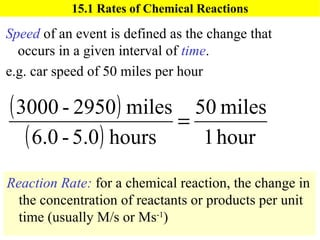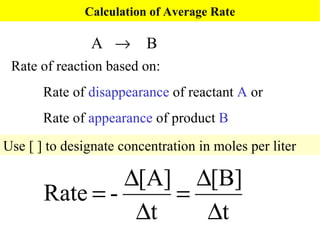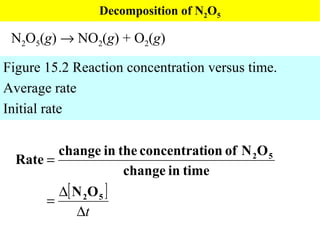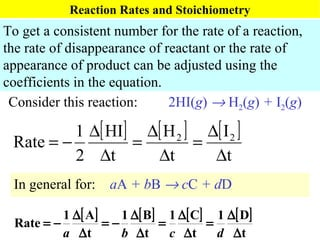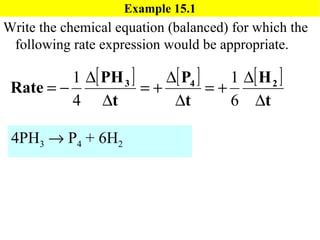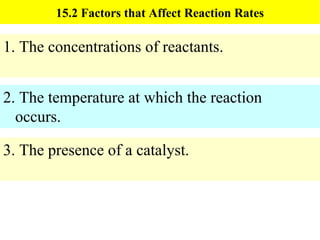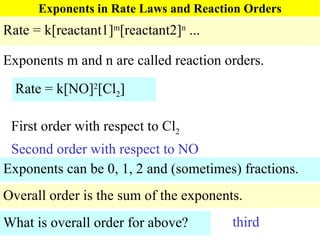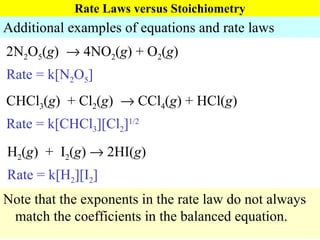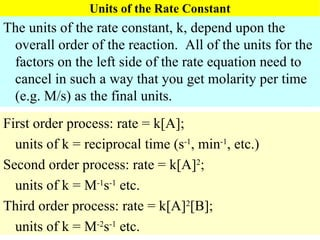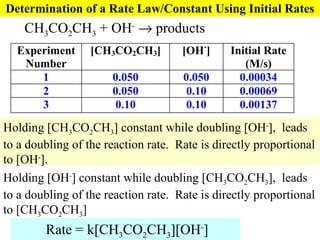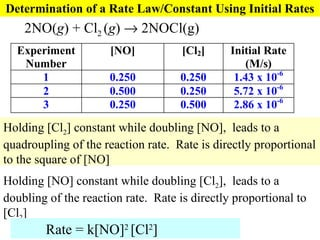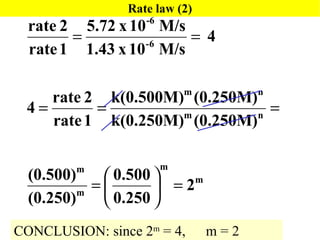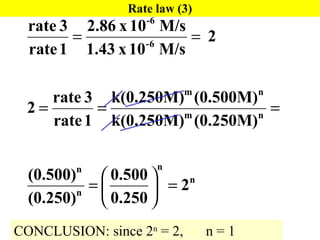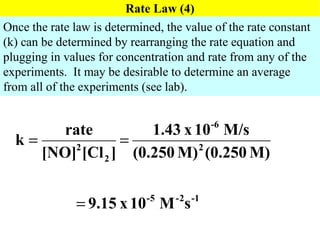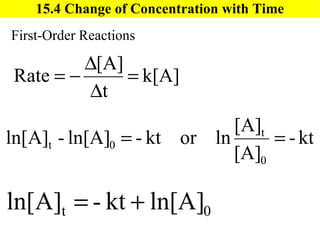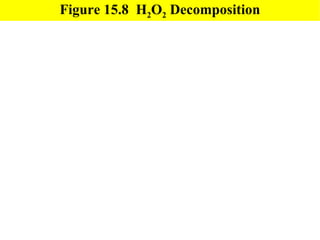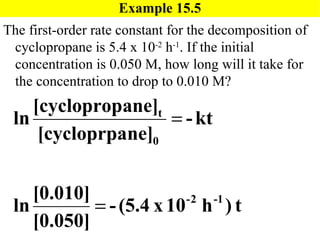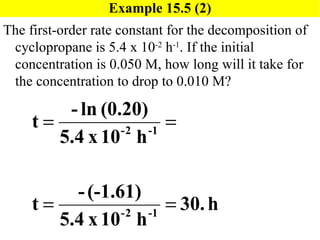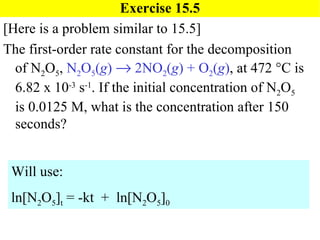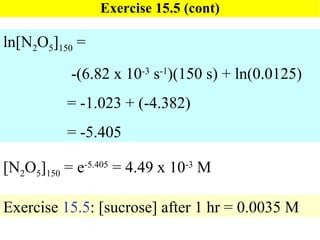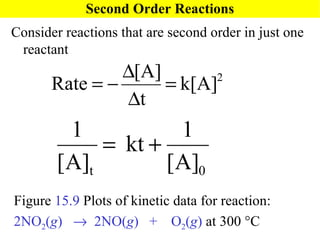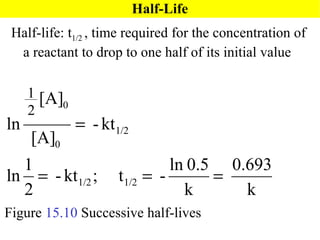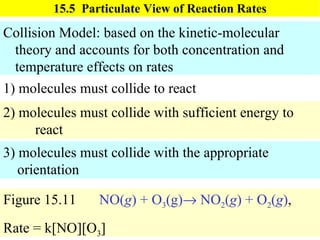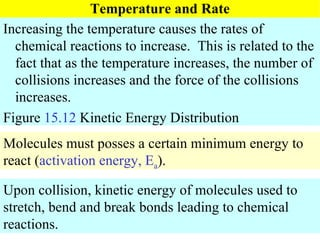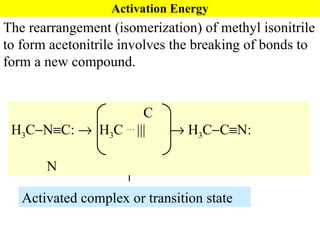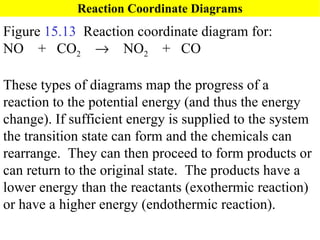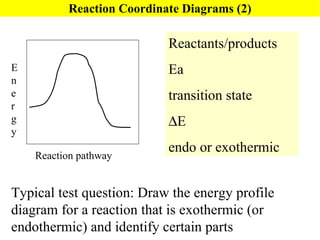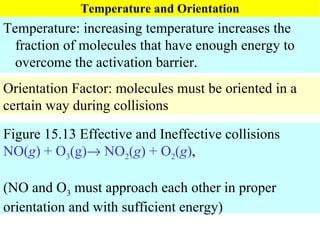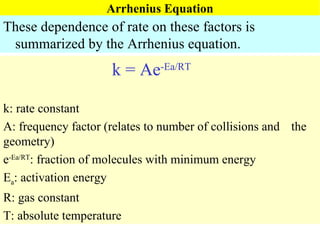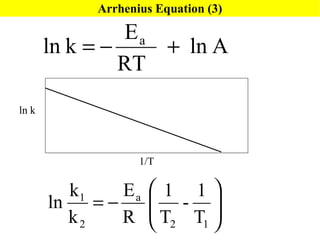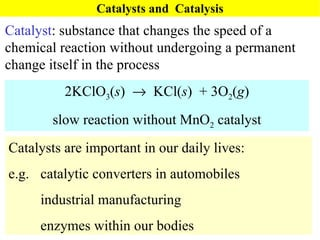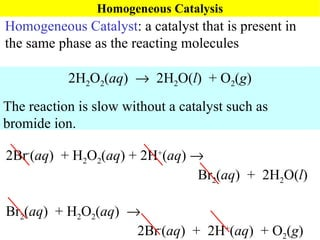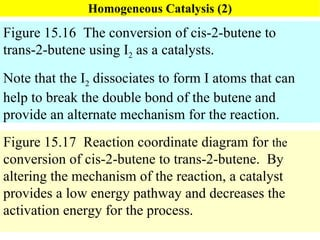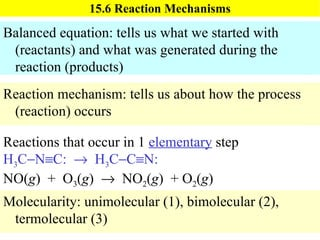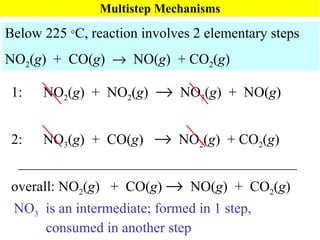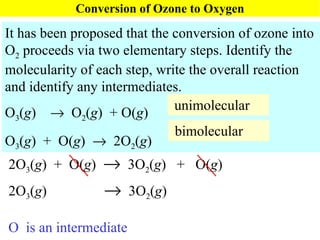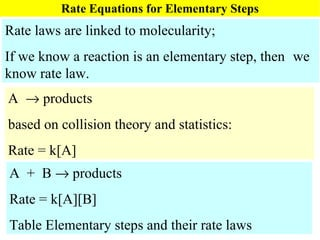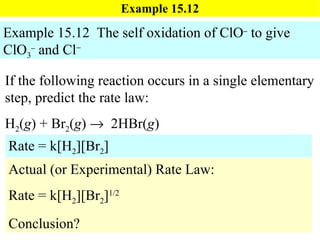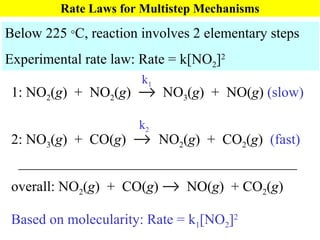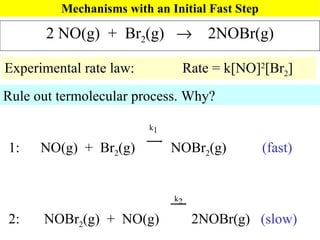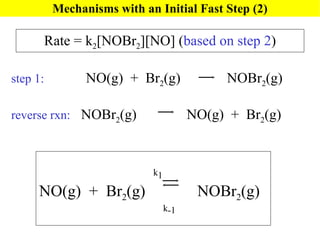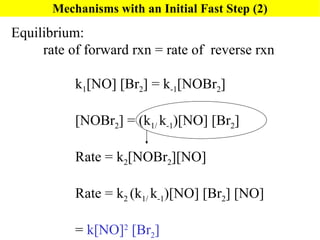1 de 50

### Ch 15 Web

• 1. CHAPTER 15 Kinetics Concerns how quickly reactions occur and the factors that affect reaction rates. Different reactions can occur at vastly different rates or speeds. Slow reactions: rusting of iron, spoiling of food Fast reactions: light triggered process such as photosynthesis ( > ms (10 -3 s) to < ps (10 -12 s)
• 2. Speed of reactions linked to mechanisms The rates or speeds of reactions are linked to their mechanisms, the detailed way in which they occur. A number of analogies can be used to help understand the concept. Consider the method of travelling from one city to another. Norfolk  Virginia Beach  direct route indirect route
• 3. Speed of reactions linked to mechanisms (2) Furthermore, the time it takes to get from one place to another depends on the method of travel. Regardless of the method, the distance between the two places is always the same. The time it takes to travel is the only thing affected by the method. Norfolk  Virginia Beach  VS
• 4. Distinguish Between Kinetics and Energetics Whether or not a chemical reaction will occur is dependent on energy changes and other factors. A decrease in energy (reactants versus products) or an increase in disorder of the system will determine whether or not a reaction will occur. Knowing that a reaction will occur tells you nothing about how quickly the reaction occurs. Thus, we tend to distinguish between kinetics and energetics . Changes in energy are associated with state functions (independent of path). Rates of reactions are highly dependent upon the mechanism by which a process takes place.
• 5. Chemical Equations and Kinetic Behavior REACTANTS  PRODUCTS C 4 H 9 Cl( aq ) + H 2 O( l )  C 4 H 9 OH( aq ) + HCl( aq ) BEFORE  AFTER
• 6. Chemical Equations and Kinetic Behavior (2) FLOUR + EGGS + WATER + OIL
• 7. Stoichiometry versus Kinetics Remember: You cannot look at the balanced equation and know how the concentration of a particular reactant will affect the rate of a reaction! 2 SLICES BREAD + 1 SLICE CHEESE = 1 SANDWICH Remember about stoichiometry, limiting reactants and yields? +  SANDWICH
• 8. Mechanisms may be simple or complex Reactions may occur in one step: e.g. A  Products or A + B  Products Some chemical reactions involve multi-step mechanisms: e.g. A + B  Products (overall reaction) mechanism: A  C C + B  Products
• 9. 15.1 Rates of Chemical Reactions Speed of an event is defined as the change that occurs in a given interval of time . e.g. car speed of 50 miles per hour Reaction Rate: for a chemical reaction, the change in the concentration of reactants or products per unit time (usually M/s or Ms -1 )
• 10. Calculation of Average Rate A  B Rate of reaction based on: Rate of disappearance of reactant A or Rate of appearance of product B Use [ ] to designate concentration in moles per liter
• 11. Decomposition of N 2 O 5 N 2 O 5 ( g )  NO 2 ( g ) + O 2 ( g ) Figure 15.2 Reaction concentration versus time. Average rate Initial rate
• 12. Reaction Rates and Stoichiometry To get a consistent number for the rate of a reaction, the rate of disappearance of reactant or the rate of appearance of product can be adjusted using the coefficients in the equation. Consider this reaction: 2HI( g )  H 2 ( g ) + I 2 ( g ) In general for: a A + b B  c C + d D
• 13. Example 15.1 Write the chemical equation (balanced) for which the following rate expression would be appropriate. 4PH 3  P 4 + 6H 2
• 14. 15.2 Factors that Affect Reaction Rates 1. The concentrations of reactants. 2. The temperature at which the reaction occurs. 3. The presence of a catalyst.
• 15. Exponents in Rate Laws and Reaction Orders Rate = k[reactant1] m [reactant2] n ... Exponents m and n are called reaction orders. Exponents can be 0, 1, 2 and (sometimes) fractions. Overall order is the sum of the exponents. What is overall order for above? third Rate = k[NO] 2 [Cl 2 ] First order with respect to Cl 2 Second order with respect to NO
• 16. Rate Laws versus Stoichiometry Additional examples of equations and rate laws 2N 2 O 5 ( g )  4NO 2 ( g ) + O 2 ( g ) Rate = k[N 2 O 5 ] CHCl 3 ( g ) + Cl 2 ( g )  CCl 4 ( g ) + HCl( g ) Rate = k[CHCl 3 ][Cl 2 ] 1/2 H 2 ( g ) + I 2 ( g )  2HI( g ) Rate = k[H 2 ][I 2 ] Note that the exponents in the rate law do not always match the coefficients in the balanced equation.
• 17. Units of the Rate Constant The units of the rate constant, k, depend upon the overall order of the reaction. All of the units for the factors on the left side of the rate equation need to cancel in such a way that you get molarity per time (e.g. M/s) as the final units. First order process: rate = k[A]; units of k = reciprocal time (s -1 , min -1 , etc.) Second order process: rate = k[A] 2 ; units of k = M -1 s -1 etc. Third order process: rate = k[A] 2 [B]; units of k = M -2 s -1 etc.
• 18. Determination of a Rate Law/Constant Using Initial Rates Holding [CH 3 CO 2 CH 3 ] constant while doubling [OH - ], leads to a doubling of the reaction rate. Rate is directly proportional to [OH - ]. Holding [OH - ] constant while doubling [CH 3 CO 2 CH 3 ], leads to a doubling of the reaction rate. Rate is directly proportional to [CH 3 CO 2 CH 3 ] Rate = k[CH 3 CO 2 CH 3 ][OH - ] CH 3 CO 2 CH 3 + OH -  products
• 19. Determination of a Rate Law/Constant Using Initial Rates Holding [Cl 2 ] constant while doubling [NO], leads to a quadroupling of the reaction rate. Rate is directly proportional to the square of [NO] Holding [NO] constant while doubling [Cl 2 ], leads to a doubling of the reaction rate. Rate is directly proportional to [Cl 2 ] Rate = k[NO] 2 [Cl 2 ] 2NO( g ) + Cl 2 ( g )  2NOCl(g)
• 20. Rate law (2) CONCLUSION: since 2 m = 4, m = 2
• 21. Rate law (3) CONCLUSION: since 2 n = 2, n = 1
• 22. Rate Law (4) Once the rate law is determined, the value of the rate constant (k) can be determined by rearranging the rate equation and plugging in values for concentration and rate from any of the experiments. It may be desirable to determine an average from all of the experiments (see lab).
• 23. 15.4 Change of Concentration with Time First-Order Reactions
• 24. Figure 15.8 H 2 O 2 Decomposition
• 25. Example 15.5 The first-order rate constant for the decomposition of cyclopropane is 5.4 x 10 -2 h -1 . If the initial concentration is 0.050 M, how long will it take for the concentration to drop to 0.010 M?
• 26. Example 15.5 (2) The first-order rate constant for the decomposition of cyclopropane is 5.4 x 10 -2 h -1 . If the initial concentration is 0.050 M, how long will it take for the concentration to drop to 0.010 M?
• 27. Exercise 15.5 [Here is a problem similar to 15.5] The first-order rate constant for the decomposition of N 2 O 5 , N 2 O 5 ( g )  2NO 2 ( g ) + O 2 ( g ) , at 472  C is 6.82 x 10 -3 s -1 . If the initial concentration of N 2 O 5 is 0.0125 M, what is the concentration after 150 seconds? Will use: ln[N 2 O 5 ] t = -kt + ln[N 2 O 5 ] 0
• 28. Exercise 15.5 (cont) [N 2 O 5 ] 150 = e -5.405 = 4.49 x 10 -3 M ln[N 2 O 5 ] 150 = -(6.82 x 10 -3 s -1 )(150 s) + ln(0.0125) = -1.023 + (-4.382) = -5.405 Exercise 15.5 : [sucrose] after 1 hr = 0.0035 M
• 29. Second Order Reactions Consider reactions that are second order in just one reactant Figure 15.9 Plots of kinetic data for reaction: 2NO 2 ( g )  2NO( g ) + O 2 ( g ) at 300  C
• 30. Half-Life Half-life: t 1/2 , time required for the concentration of a reactant to drop to one half of its initial value Figure 15.10 Successive half-lives
• 31. 15.5 Particulate View of Reaction Rates Collision Model: based on the kinetic-molecular theory and accounts for both concentration and temperature effects on rates 1) molecules must collide to react 3) molecules must collide with the appropriate orientation 2) molecules must collide with sufficient energy to react Figure 15.11 NO( g ) + O 3 (g)  NO 2 ( g ) + O 2 ( g ) , Rate = k[NO][O 3 ]
• 32. Temperature and Rate Increasing the temperature causes the rates of chemical reactions to increase. This is related to the fact that as the temperature increases, the number of collisions increases and the force of the collisions increases. Figure 15.12 Kinetic Energy Distribution Molecules must posses a certain minimum energy to react ( activation energy, E a ). Upon collision, kinetic energy of molecules used to stretch, bend and break bonds leading to chemical reactions.
• 33. Activation Energy The rearrangement (isomerization) of methyl isonitrile to form acetonitrile involves the breaking of bonds to form a new compound. Activated complex or transition state C H 3 C  N  C:  H 3 C … |||  H 3 C  C  N: N
• 34. Reaction Coordinate Diagrams Figure 15.13 Reaction coordinate diagram for: NO + CO 2  NO 2 + CO These types of diagrams map the progress of a reaction to the potential energy (and thus the energy change). If sufficient energy is supplied to the system the transition state can form and the chemicals can rearrange. They can then proceed to form products or can return to the original state. The products have a lower energy than the reactants (exothermic reaction) or have a higher energy (endothermic reaction).
• 35. Reaction Coordinate Diagrams (2) Typical test question: Draw the energy profile diagram for a reaction that is exothermic (or endothermic) and identify certain parts Reactants/products Ea transition state  E endo or exothermic Energy Reaction pathway
• 36. Temperature and Orientation Temperature: increasing temperature increases the fraction of molecules that have enough energy to overcome the activation barrier. Orientation Factor: molecules must be oriented in a certain way during collisions Figure 15.13 Effective and Ineffective collisions NO( g ) + O 3 (g)  NO 2 ( g ) + O 2 ( g ) , (NO and O 3 must approach each other in proper orientation and with sufficient energy)
• 37. Arrhenius Equation These dependence of rate on these factors is summarized by the Arrhenius equation. k = Ae -Ea/RT k: rate constant A: frequency factor (relates to number of collisions and the geometry) e -Ea/RT : fraction of molecules with minimum energy E a : activation energy R: gas constant T: absolute temperature
• 38. Arrhenius Equation (3) ln k 1/T
• 39. Catalysts and Catalysis Catalyst : substance that changes the speed of a chemical reaction without undergoing a permanent change itself in the process 2KClO 3 ( s )  KCl( s ) + 3O 2 ( g ) slow reaction without MnO 2 catalyst Catalysts are important in our daily lives: e.g. catalytic converters in automobiles industrial manufacturing enzymes within our bodies
• 40. Homogeneous Catalysis Homogeneous Catalyst : a catalyst that is present in the same phase as the reacting molecules 2H 2 O 2 ( aq )  2H 2 O( l ) + O 2 ( g ) The reaction is slow without a catalyst such as bromide ion. 2Br - ( aq ) + H 2 O 2 ( aq ) + 2H + ( aq )  Br 2 ( aq ) + 2H 2 O( l ) Br 2 ( aq ) + H 2 O 2 ( aq )  2Br - ( aq ) + 2H + ( aq ) + O 2 ( g )
• 41. Homogeneous Catalysis (2) Figure 15.16 The conversion of cis-2-butene to trans-2-butene using I 2 as a catalysts. Note that the I 2 dissociates to form I atoms that can help to break the double bond of the butene and provide an alternate mechanism for the reaction. Figure 15.17 Reaction coordinate diagram for the conversion of cis-2-butene to trans-2-butene. By altering the mechanism of the reaction, a catalyst provides a low energy pathway and decreases the activation energy for the process.
• 42. 15.6 Reaction Mechanisms Balanced equation: tells us what we started with (reactants) and what was generated during the reaction (products) Reaction mechanism: tells us about how the process (reaction) occurs Reactions that occur in 1 elementary step H 3 C  N  C:  H 3 C  C  N: NO( g ) + O 3 ( g )  NO 2 ( g ) + O 2 ( g ) Molecularity: unimolecular (1), bimolecular (2), termolecular (3)
• 43. Multistep Mechanisms 1: NO 2 ( g ) + NO 2 ( g )  NO 3 ( g ) + NO( g ) 2: NO 3 ( g ) + CO( g )  NO 2 ( g ) + CO 2 ( g ) _______________________________________ overall: NO 2 ( g ) + CO( g )  NO( g ) + CO 2 ( g ) Below 225  C, reaction involves 2 elementary steps NO 2 ( g ) + CO( g )  NO( g ) + CO 2 ( g ) NO 3 is an intermediate; formed in 1 step, consumed in another step
• 44. Conversion of Ozone to Oxygen It has been proposed that the conversion of ozone into O 2 proceeds via two elementary steps. Identify the molecularity of each step, write the overall reaction and identify any intermediates. O 3 ( g )  O 2 ( g ) + O( g ) O 3 ( g ) + O( g )  2O 2 ( g ) unimolecular O is an intermediate bimolecular 2O 3 ( g ) + O( g )  3O 2 ( g ) + O( g ) 2O 3 ( g )  3O 2 ( g )
• 45. Rate Equations for Elementary Steps Rate laws are linked to molecularity; If we know a reaction is an elementary step, then we know rate law. A  products based on collision theory and statistics: Rate = k[A] A + B  products Rate = k[A][B] Table Elementary steps and their rate laws
• 46. Example 15.12 If the following reaction occurs in a single elementary step, predict the rate law: H 2 ( g ) + Br 2 ( g )  2HBr( g ) Rate = k[H 2 ][Br 2 ] Actual (or Experimental) Rate Law: Rate = k[H 2 ][Br 2 ] 1/2 Conclusion? Example 15.12 The self oxidation of ClO  to give ClO 3  and Cl 
• 47. Rate Laws for Multistep Mechanisms Below 225  C, reaction involves 2 elementary steps Experimental rate law: Rate = k[NO 2 ] 2 Based on molecularity: Rate = k 1 [NO 2 ] 2 1: NO 2 ( g ) + NO 2 ( g )  NO 3 ( g ) + NO( g ) (slow) 2: NO 3 ( g ) + CO( g )  NO 2 ( g ) + CO 2 ( g ) (fast) _______________________________________ overall: NO 2 ( g ) + CO( g )  NO( g ) + CO 2 ( g ) k 1 k 2
• 48. Mechanisms with an Initial Fast Step 2 NO(g) + Br 2 (g)  2NOBr(g) Experimental rate law: Rate = k[NO] 2 [Br 2 ] Rule out termolecular process. Why? k 1 1: NO(g) + Br 2 (g) NOBr 2 (g) (fast) k 2 2: NOBr 2 (g) + NO(g) 2NOBr(g) (slow)
• 49. Mechanisms with an Initial Fast Step (2) Rate = k 2 [NOBr 2 ][NO] ( based on step 2 ) step 1: NO(g) + Br 2 (g) NOBr 2 (g) reverse rxn: NOBr 2 (g) NO(g) + Br 2 (g) k 1 NO(g) + Br 2 (g) NOBr 2 (g) k -1
• 50. Mechanisms with an Initial Fast Step (2) Equilibrium: rate of forward rxn = rate of reverse rxn k 1 [NO] [Br 2 ] = k -1 [NOBr 2 ] [NOBr 2 ] = (k 1/ k -1 )[NO] [Br 2 ] Rate = k 2 [NOBr 2 ][NO] Rate = k 2 (k 1/ k -1 )[NO] [Br 2 ] [NO] = k[NO] 2 [Br 2 ]

### Notas del editor

1. chemical kinetics : speed of reactions, desire to know, understand speed up: synthesis rxns, explosions slow down: corrosion, spoiling control: biochemical processes speed depends on mechanism logistical models , analogies used to help conceptualize what factors influence rates etc Pizza eating example: - have pizza party, monitor how long it takes class to consume pizza - observe number of empty boxes; note to monitor chem rxn must monitor 1 or more reactants/products; may not know details of what is going on Results : count 3 boxes; express result a variety of ways (each conveys some number that expresses the rate of consumption of pizza)
2. chemical kinetics : speed of reactions, desire to know, understand speed up: synthesis rxns, explosions slow down: corrosion, spoiling control: biochemical processes speed depends on mechanism logistical models , analogies used to help conceptualize what factors influence rates etc Pizza eating example: - have pizza party, monitor how long it takes class to consume pizza - observe number of empty boxes; note to monitor chem rxn must monitor 1 or more reactants/products; may not know details of what is going on Results : count 3 boxes; express result a variety of ways (each conveys some number that expresses the rate of consumption of pizza)
3. chemical kinetics : speed of reactions, desire to know, understand speed up: synthesis rxns, explosions slow down: corrosion, spoiling control: biochemical processes speed depends on mechanism logistical models , analogies used to help conceptualize what factors influence rates etc Pizza eating example: - have pizza party, monitor how long it takes class to consume pizza - observe number of empty boxes; note to monitor chem rxn must monitor 1 or more reactants/products; may not know details of what is going on Results : count 3 boxes; express result a variety of ways (each conveys some number that expresses the rate of consumption of pizza)
4. chemical kinetics : speed of reactions, desire to know, understand speed up: synthesis rxns, explosions slow down: corrosion, spoiling control: biochemical processes speed depends on mechanism logistical models , analogies used to help conceptualize what factors influence rates etc Pizza eating example: - have pizza party, monitor how long it takes class to consume pizza - observe number of empty boxes; note to monitor chem rxn must monitor 1 or more reactants/products; may not know details of what is going on Results : count 3 boxes; express result a variety of ways (each conveys some number that expresses the rate of consumption of pizza)